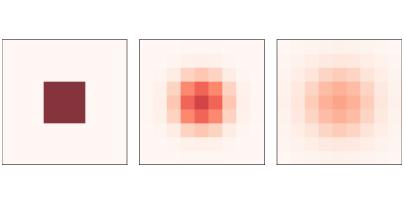# 9.2. Diffusion¶

In 1952 Alan Turing published a paper called “The chemical basis of morphogenesis”, which describes the behavior of systems involving two chemicals that diffuse in space and react with each other. He showed that these systems produce a wide range of patterns, depending on the diffusion and reaction rates, and conjectured that systems like this might be an important mechanism in biological growth processes, particularly the development of animal coloration patterns.

Turing’s model is based on differential equations, but it can be implemented using a cellular automaton.

Before we get to Turing’s model, we’ll start with something simpler: a diffusion system with just one chemical. We’ll use a 2-D CA where the state of each cell is a continuous quantity (usually between 0 and 1) that represents the concentration of the chemical.

We’ll model the diffusion process by comparing each cell with the average of its neighbors. If the concentration of the center cell exceeds the neighborhood average, the chemical flows from the center to the neighbors. If the concentration of the center cell is lower, the chemical flows the other way.

The following kernel computes the difference between each cell and the average of its neighbors:

```kernel = np.array([[0, 1, 0],
[1,-4, 1],
[0, 1, 0]])
```

Using `np.correlate2d`, we can apply this kernel to each cell in an array:

```c = correlate2d(array, kernel, mode='same')
```

We’ll use a diffusion constant, `r`, that relates the difference in concentration to the rate of flow:

```array += r * c
```Figure 9.1: A simple diffusion model after 0, 5, and 10 steps.

Figure 9.1 shows results for a CA with size `n=9`, diffusion constant `r=0.1`, and initial concentration 0 everywhere except for an “island” in the middle. The figure shows the starting configuration and the state of the CA after 5 and 10 steps. The chemical spreads from the center outward, continuing until the concentration is the same everywhere.